## Excel tutorial – extract text or number by specific position

In many cases, you may only need to extract the useful content from a sentence or a text string in a cell, such as extracting the province from the address, extracting the email address from a sentence, extracting the courier bill number from a conversation, and so on. This tutorial narrows down the extraction to a specific position in a cell and collects different methods to help extract text or numbers from a cell by specific position in Excel.

#### Extract text by position

This section gathers common positions where a text can be extracted from a cell and provides corresponding methods to handle them step-by-step. You can browse for more details.

##### 1. Extract the number of characters from left or right

To extract the number of characters from the left or right side of a string, you can try one of the below methods.

1.1 Extract the first or last N characters with formulas

Supposing you have a text string list in column B as shown in the screenshot below, to extract the first 2 characters and the last 2 characters from each string, you can apply the following formulas.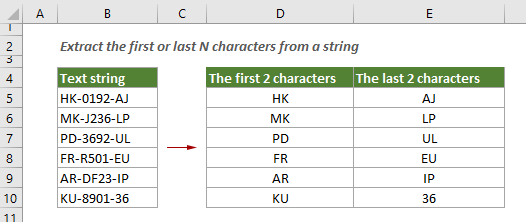Extract the first N characters from a text string

The LEFT function can help to easily extract the first N characters from a text string in Excel.

Generic formula

=LEFT(text_string,[num_chars])

Arguments

Text_string: The text string that contains the characters you want to extract. It can be a cell reference or an actual text string enclosed in double quotation marks;
Num_chars: Specifies the number of characters you want to extract.
The Num_chars must be greater than or equal to zero;
If the specified Num_chars is greater than the length of the text string, it returns the whole text;
If The Num_chars is omitted, it is assumed to be 1.

Now you can apply this formula to extract the first 2 characters from cells in column B.

1. Select a blank cell, copy or enter the formula below and press the Enter key to get the first result. Select the result cell and drag its AutoFill Handle down to apply the formula to other cells.

=LEFT(B5,2)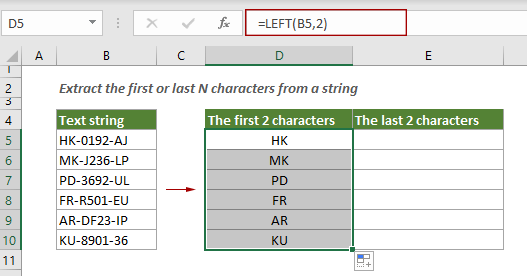Now the first 2 characters in each cell of range B5:B10 have been extracted.

Extract the last N characters from a text string

Here we apply the RIGHT function to extract the last N characters from a text string in Excel.

Generic formula

=RIGHT(text_string,[num_chars])

Arguments

Text_string: The text string that contains the characters you want to extract. It can be a cell reference or an actual text string enclosed in double quotation marks;
Num_chars: Specifies the number of characters you want to extract.
The Num_chars must be greater than or equal to zero;
If the specified Num_chars is greater than the length of the text string, it returns the whole text;
If The Num_chars is omitted, it is assumed to be 1.

Select a blank cell, copy or enter the formula below and press the Enter key to get the result. Then select this result cell and drag its AutoFill Handle down to get other results.

=RIGHT(B5,2)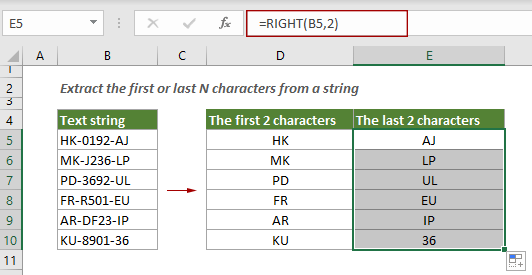1.2 Extract the first or last N characters with an amazing tool

Although the formulas above are simple, to extracting the first or last n characters from a long list of text string, you still need to drag the AutoFill Handle from top to bottom which maybe a little time-consuming. Here recommends Kutools for Excel’s Extract Text utility to help extract the first or last N characters from a list of text string in bulk.

1. Select the text string list you want to extract text from in advance and click Kutools > Text > Extract Text.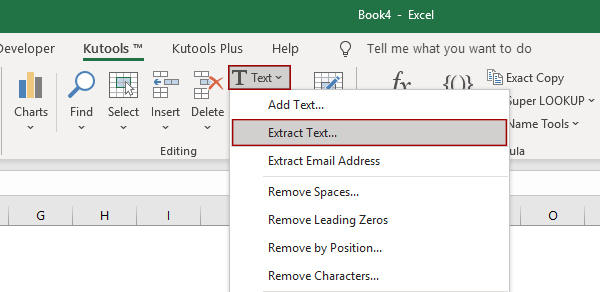2. In the popping up Extract Text dialog box, you need to configure as follows.

2.1) Ensure you are staying in the Extract by location tab;
2.2) In the Range box, the selected range is displayed inside, you can change to another range according to your needs;
2.3) In the Options section:
If you want to extract the first N characters, choose the The first N character radio button, and then specify the number of character you will extract in the text box. In this case, I enter the number 2;
If you want to extract the last N characters, select the The last N character radio button, and then specify the number of character you will extract in the text box. In this case, I want to extract the last 2 characters from the text string, so I enter the number 2 in the text box.
2.4) Click OK. See screenshot: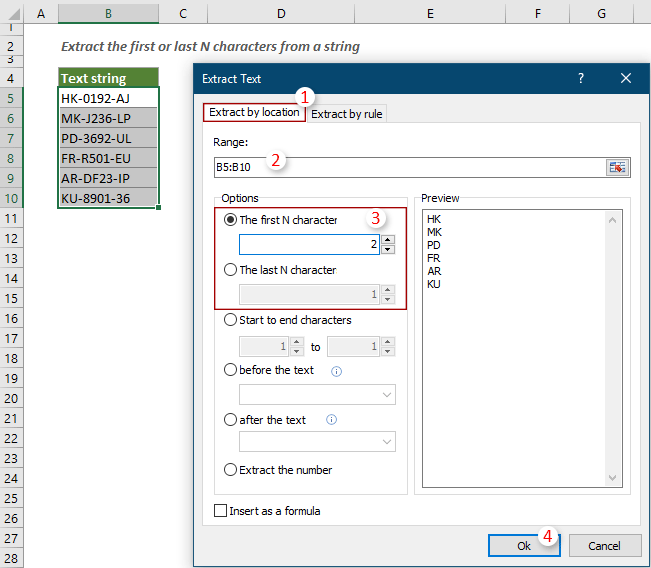Note: To make the result dynamic when the text string changes, you can check the Insert as a formula box.

3. In the next popping up Extract Text dialog box, choose a cell to output the extracted characters and then click OK.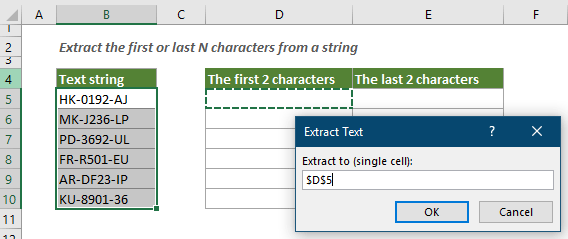Then the specified first or last N characters are extracted from selected cells in bulk.

If you want to have a free trial (30-day) of this utility, please click to download it, and then go to apply the operation according above steps.

##### 2. Extract text before or after a certain character/word

To extract text before or after a certain character or word, the different scenarios in this section will meet your needs.

2.1 Extract text before or after the first delimiter (a character)

As shown in the screenshot below, to extract text before or after the first delimiter from each cell in the range B4:B10, you can apply one of the below methods.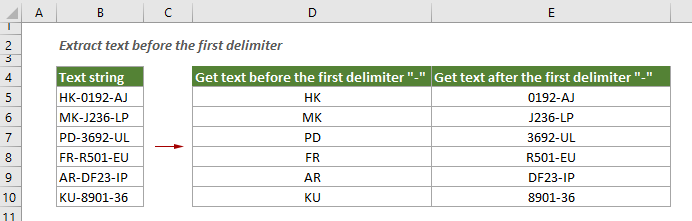2.1.1 Extract text before the first delimiter with formula

Apply a formula based on the LEFT and FIND functions can help to extract text before the first delimiter from a cell. You can follow the steps below to get it done.

Generic formula

=LEFT(text_string,FIND("delimiter",text_string,1)-1)

Arguments

Text_string: The text string from which you want to extract the substring. It can be a cell reference or an actual text string enclosed in double quotation marks;
Delimiter: The first delimiter that defines which text will be extracted from a cell.

Select a blank cell, copy or enter the formula below into it and press the Enter key to get the first result. Select the first result cell and drag its AutoFill Handle down to get the texts before the first delimiter of other cells.

=LEFT(B5,FIND("-",B5,1)-1)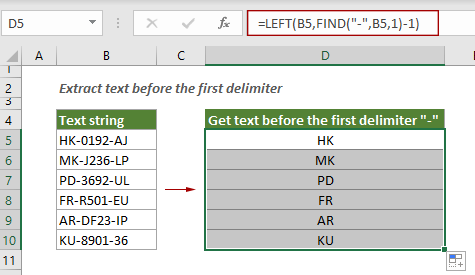2.1.2 Extract text after the first delimiter with formula

The below formula helps to extract text after the first delimiter from a cell in Excel.

Generic formula

=MID(text_string,FIND("delimiter",text_string)+1,LEN(text_string))

Arguments

Text_string: The text string from which you want to extract substring. It can be a cell reference or an actual text string enclosed in double quotation marks;
Delimiter: The first delimiter that defines which text will be extracted from a cell.
Select a blank cell, copy or enter the formula below into it and press the Enter key to get the first result. Select the first result cell and drag its AutoFill Handle down to get the other results.

=MID(B5,FIND("-",B5)+1,LEN(B5))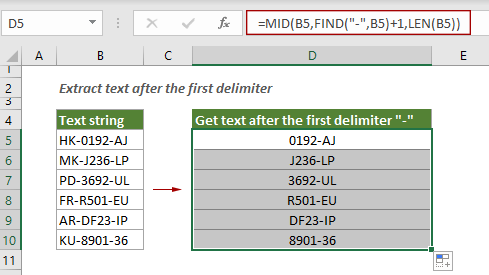2.1.3 Extract text before or after the first delimiter with an amazing tool

Here highly recommends the Extract Text utility of Kutools for Excel. With this feature, you can easily extract texts before or after the first delimiter from a range of cells in bulk.

1. Select the range of cells where you want to extract the text, and then click Kutools > Text > Extract Text.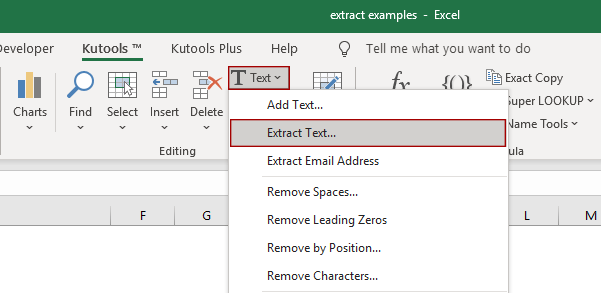2. In the Extract Text dialog box, you need to configure as follows.

2.1) Stay in the Extract by location tab;
2.2) In the Range box, the selected range is displayed inside, you can change it as you need;
2.3) In the Options section:
To extract text before the first delimiter, select the before the text radio button, and then type the first delimiter into the text box;
To extract text after the first delimiter, select the after the text radio button, and then enter the first delimiter into the text box.
2.4) Click OK.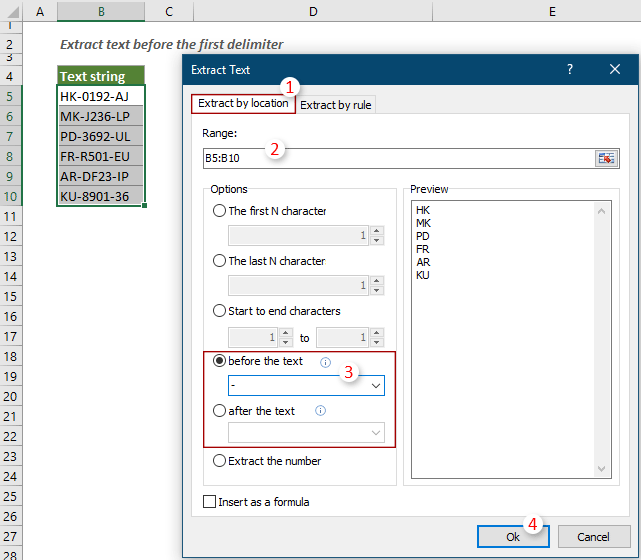Note: To make the result dynamic when the text string changes, you can check the Insert as a formula box.

3. Then another Extract Text dialog box pops up, choose a cell to output the results and click OK.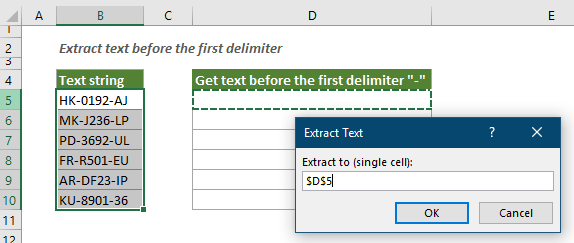Then texts before or after the first delimiter are extracted from selected cells at once.

If you want to have a free trial (30-day) of this utility, please click to download it, and then go to apply the operation according above steps.

2.2 Extract text before or after the last delimiter (a character)

In the steps above we have learned the methods about extracting text before or after the first delimiter from a cell. As shown in the screenshot below, this section will show you two formulas to extract text before or after the last delimiter from a cell. You can follow the steps below to get it done.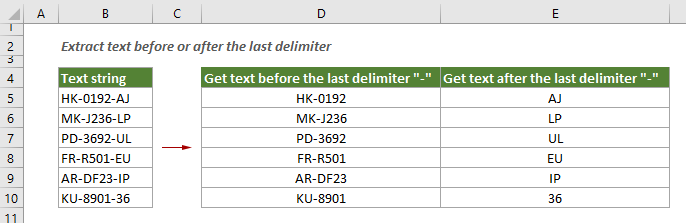2.2.1 Extract text before the last delimiter with formula

To extract text before the last delimiter from a cell, you can use the SEARCH, LEN and SUBSTITUTE functions inside of the LEFT function.

Generic formula

=LEFT(text_string,SEARCH("#",SUBSTITUTE(text_string,"delimiter","#",LEN(text_string)-LEN(SUBSTITUTE(text_string,"delimiter",""))))-1)

Arguments

Text_string: The text string from which you want to extract the substring. It can be a cell reference or an actual text string enclosed in double quotation marks;
Delimiter: The last delimiter that defines which text will be extracted from a cell.

Select a cell, enter the formula below and press the Enter key to get the result. Select this result cell and drag its AutoFill Handle down to extract the texts from other text strings in the same column.

=LEFT(B5,SEARCH("#",SUBSTITUTE(B5,"-","#",LEN(B5)-LEN(SUBSTITUTE(B5,"-",""))))-1)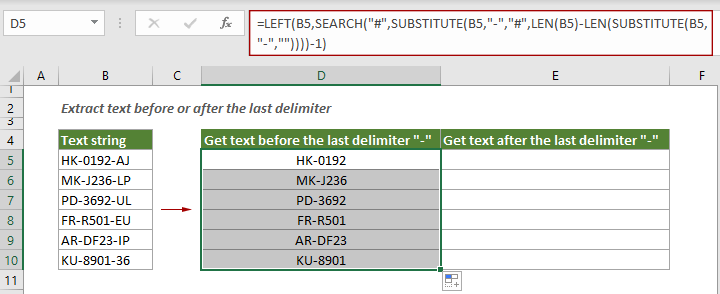2.2.2 Extract text after the last delimiter with formula

After extracting text before the last delimiter from a cell, you can apply the formula below to extract the text after the last delimiter as you need.

Generic formula

=RIGHT(text_string,LEN(text_string)-SEARCH("#",SUBSTITUTE(text_string,"delimiter","#",LEN(text_string)-LEN(SUBSTITUTE(text_string,"delimiter","")))))

Arguments

Text_string: The text string from which you want to extract the substring. It can be a cell reference or an actual text string enclosed in double quotation marks;
Delimiter: The last delimiter that defines which text will be extracted from a cell.

Select a cell, enter the formula below and press the Enter key to get the result. Select this result cell and drag its AutoFill Handle down to extract the texts from other text strings in the same column.

=RIGHT(B5,LEN(B5)-SEARCH("#",SUBSTITUTE(B5,"-","#",LEN(B5)-LEN(SUBSTITUTE(B5,"-","")))))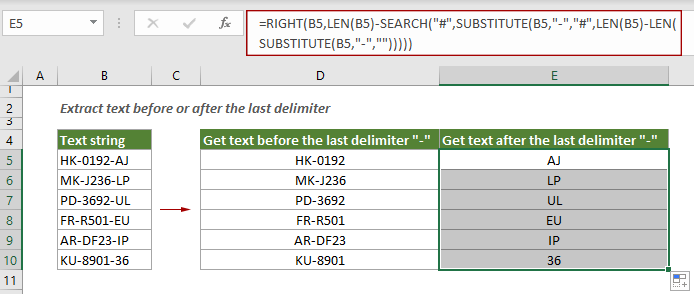2.3 Extract text after the nth character

Look at the example below, there is a list of text string in the range B4:B10, to extract the text after the third character from each cell, you can apply a formula based on the MID function and the LEN function.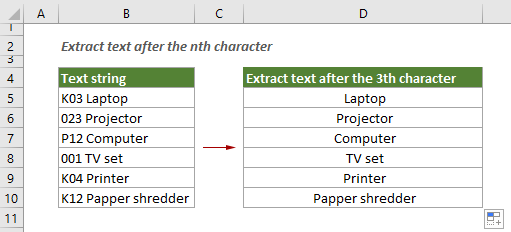Generic formula

=MID(text_string,nth_char+1,LEN(text_string))

Arguments

Text_string: The text string from which you want to extract the substring. It can be a cell reference or an actual text string enclosed in double quotation marks;
nth_char: A number represents the nth character, and you will extract the text after it.

Select a blank cell, copy or enter the formula below into it and press the Enter key to get the result. Select this result cell and drag its AutoFill Handle down to get other results.

=MID(B5,3+1,LEN(B5))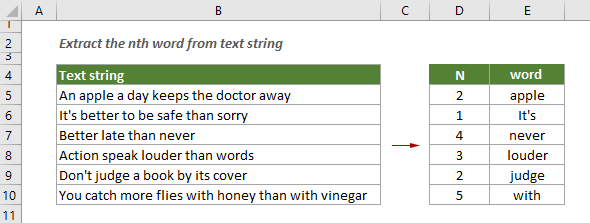2.4 Extract the nth word from a text string

Supposing you have a list of text string as shown in the screenshot below, and want to extract only the nth word from the text string, this section provides three methods for you to get it done.

2.4.1 Extract the nth word with formula

You can combine the TRIM, MID, SUBSTITUTE, REPT and LEN functions to extract the nth word from a text string in a cell.

Generic formula

=TRIM(MID(SUBSTITUTE(text_string," ",REPT(" ",LEN((text_string))), (N-1)*LEN((text_string)+1, LEN((text_string)))

Arguments

Text_string: The text string from which you want to extract the nth word. It can be a cell reference or an actual text string enclosed in double quotation marks;
N: A number represents the nth word you will extract from the text string.

In this case, the range B5:B10 contains the text strings, D5:D10 contains the numbers representing the nth word, let’s apply this formula to extract the nth word from text string.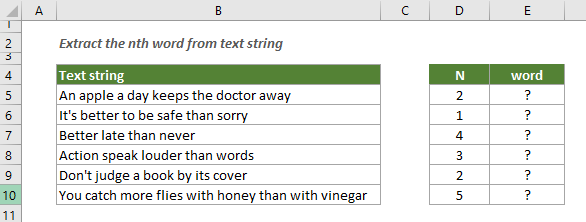Select a blank cell, copy or enter the formula below into it and press the Enter key to get the first result. Select this result cell and drag its AutoFill Handle down to get the nth word of other cells.

=TRIM(MID(SUBSTITUTE(B5," ",REPT(" ",LEN(B5))), (D5-1)*LEN(B5)+1, LEN(B5)))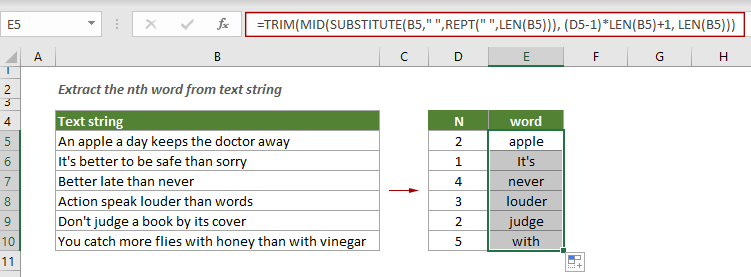Note: You can directly type the nth number in the formula as follows.

=TRIM(MID(SUBSTITUTE(B5," ",REPT(" ",LEN(B5))), (2-1)*LEN(B5)+1, LEN(B5)))

2.4.2 Extract the nth word with User-defined function

Apart from the above formula, you can also apply a user-defined function to extract the nth word from a cell in Excel.

1. Press the Alt + F11 keys to open the Microsoft Visual Basic for Applications window.

2. In the Microsoft Visual Basic for Applications window, click Insert > Module, and then copy the VBA below into the Code window.

VBA code: Extract the nth word from a text string in a cell

``````Function ExtractTheNthWord(Source As String, Position As Integer)
'Update by Extendoffice 20211202
Dim arr() As String
arr = VBA.Split(Source, " ")
xCount = UBound(arr)
If xCount < 1 Or (Position - 1) > xCount Or Position < 0 Then
FindWord = ""
Else
FindWord = arr(Position - 1)
End If
End Function``````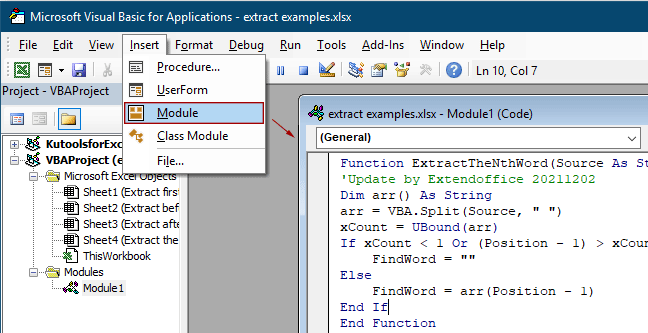3. Press the Alt + Q keys to close the Microsoft Visual Basic for Applications window.

4. Go back to the worksheet containing the text strings which you want to extract the nth word from. Select a blank cell, copy or enter the formula below into it and press the Enter key to get the nth word.

=FindWord(B5,D5)

Or

=FindWord(B5,2)

Note: in the formula, D5 is the cell that contains a number representing the nth word. Alternatively, you can directly replace the cell reference with a number.

5. Select the result cell and drag its AutoFill Handle down to extract the nth word from the text strings of other cells.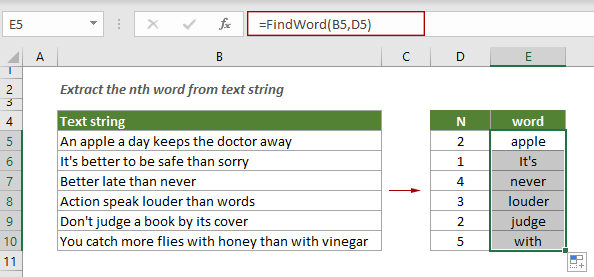2.4.3 Extract the nth word with an amazing tool

If you don’t want to manually apply a formula or a user-defined function provided above, here recommends Kutools for Excel’s Extract the nth word in cell utility. With this feature, you can easily extract the nth word from a text string in a cell with a few clicks only.

1. Select a cell to place the result, and click Kutools > Formula Helper > Text > Extract the nth word in cell. See screenshot: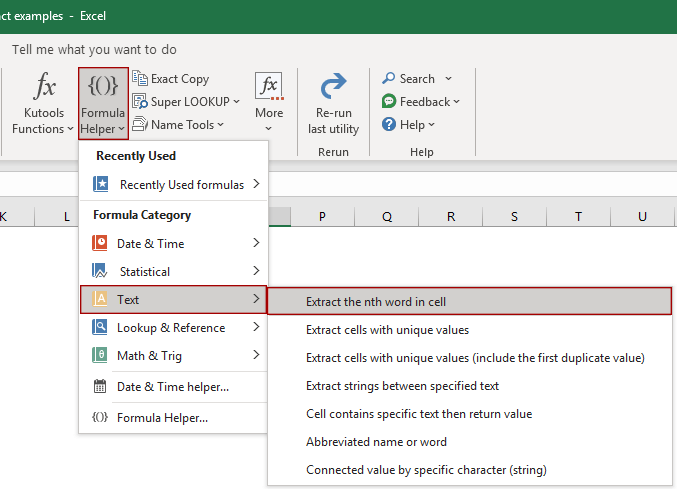2. In the Formulas Helper dialog box, you need to configure as follows.

2.1) In the Choose a formula list box, the Extract the nth word in cell option is highlighted;
2.2) In the Cell box, choose a cell contains the text string which you want to extract the nth word from;
2.3) In the The Nth box, select a cell containing the nth number or directly enter a number according to your needs;
2.4) Click OK.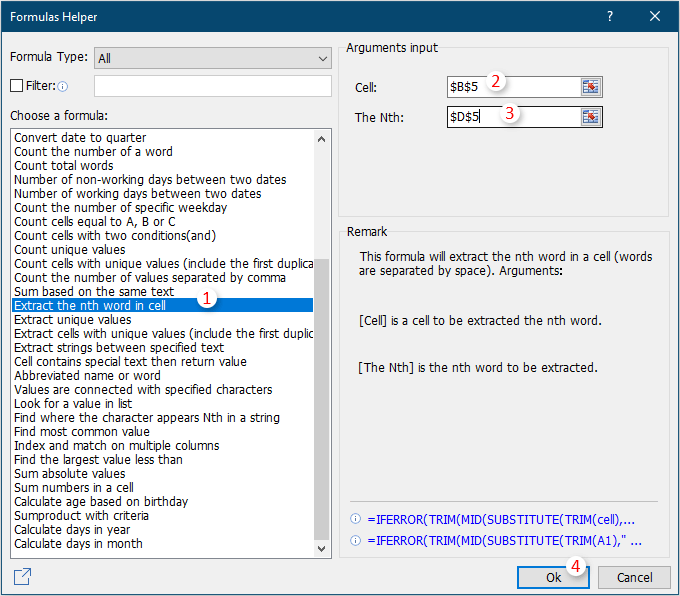3. Then the nth (second) word is extracted from the text string in the cell B5, and you can see a formula is created at the same time. Select this result cell and drag its AutoFill Handle down to get the nth word from other text strings.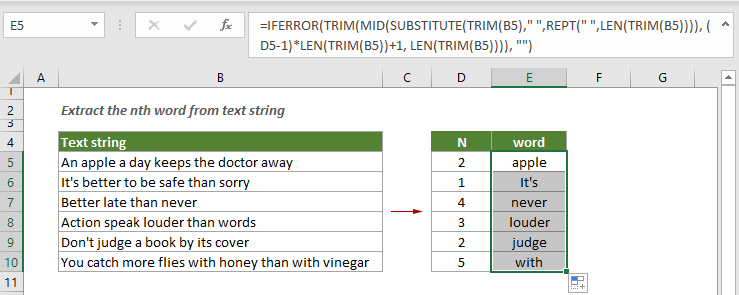If you want to have a free trial (30-day) of this utility, please click to download it, and then go to apply the operation according above steps.

2.5 Extract text before or after the nth occurrence of a delimiter

Supposing you have a list of text strings as shown in the screenshot below. To extract the text before or after the second occurrence of a space, this section provides two formulas to help you get it done.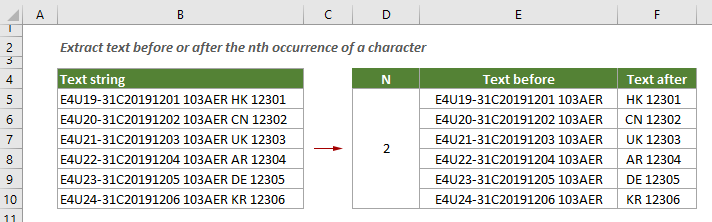2.5.1 Extract text before the nth occurrence of a delimiter

You can use the LEFT function along with the SUBSTITUTE and FIND functions to extract text before the nth occurrence of a delimiter from a cell in Excel.

Generic formula

=LEFT(SUBSTITUTE(text_string,"delimiter",CHAR(9),n),FIND(CHAR(9),SUBSTITUTE(text_string,"delimiter",CHAR(9),n),1)-1)

Arguments

Text_string: The text string from which you want to extract the text. It can be a cell reference or an actual text string enclosed in double quotation marks;
N: A number represents the nth occurrence of a delimiter which you will extract text before it.

Select a cell, copy or enter the formula below and press the Enter key to get the result. Select this result cell and drag it AutoFill Handle down to get other results in the list.

=LEFT(SUBSTITUTE(B5," ",CHAR(9),2),FIND(CHAR(9),SUBSTITUTE(B5," ",CHAR(9),2),1)-1)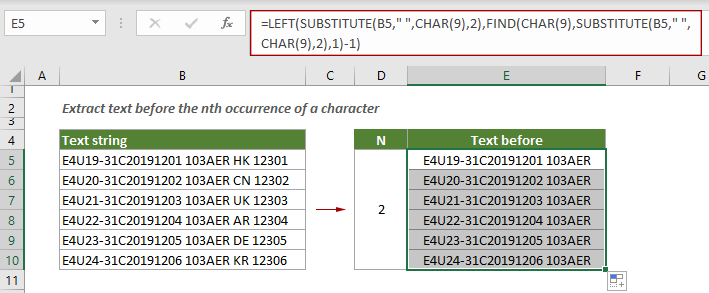Note: In the formula, B5 is the cell containing the text string from which you want to extract the text; “ ” here represents a space and the number 2 represents the second occurrence of a space. You can change them according to your needs.

2.5.2 Extract text after the nth occurrence of a delimiter

To extract text after the nth occurrence of a delimiter, you can apply the RIGHT function with the SUBSTITUTE, LEN and FIND functions.

Generic formula

=RIGHT(SUBSTITUTE(text_string, "delimiter", CHAR(9), n), LEN(text_string)- FIND(CHAR(9), SUBSTITUTE(text_string, "delimiter", CHAR(9), n), 1) + 1)

Arguments

Text_string: The text string from which you want to extract the text. It can be a cell reference or an actual text string enclosed in double quotation marks;
N: A number represents the nth occurrence of a delimiter which you will extract text after it.

Now you can apply this formula to extract the text after the second occurrence of the space from each cell in range B5:B10 as follows.

Select a cell, enter the formula below and press the Enter key to get the result. Select this result cell and drag it AutoFill Handle down to get other results.

=RIGHT(SUBSTITUTE(B5, " ", CHAR(9), 2), LEN(B5)- FIND(CHAR(9), SUBSTITUTE(B5, " ", CHAR(9), 2), 1) + 1)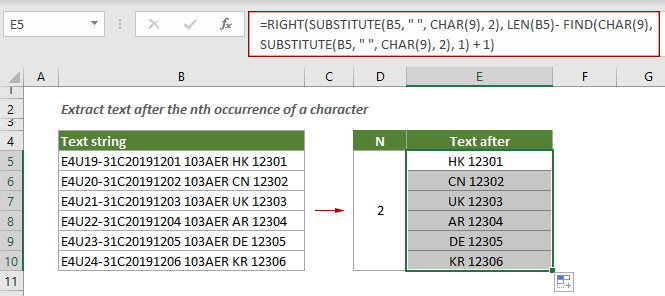2.6 Extract text before or after line break

Suppose you have an order list in column B and you want to extract only the date part and the product No. part from each cell. You can use the below Excel formulas to get it done.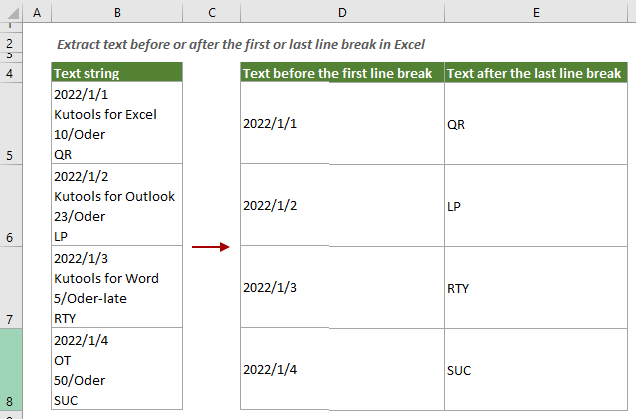2.6.1 Extract text before the first line break with formula

As you can see in the screenshot above, the date part locates before the first line break within the cell. This section will demonstrate a LEFT function along with the SEARCH function to help you extract the text before the first line break within a cell.

Generic formula

=LEFT(cell, SEARCH(CHAR(10), cell)-1)

Arguments

Cell: The cell from which you want to extract the text before the first line break.

Select a blank cell, copy or enter the formula below into it and press the Enter key to get the result. Select this result cell and then drag its AutoFill Handle down to apply this formula to other cells.

=LEFT(B5, SEARCH(CHAR(10), B5)-1)

Then you can see the text before the first line break in each cell in range B5:B8 is extracted as shown in the screenshot below.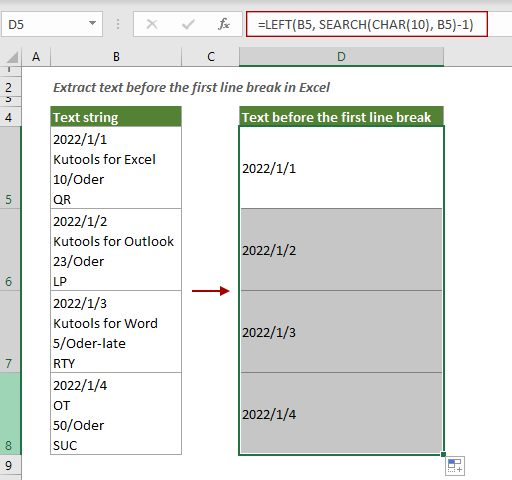Note: In the formula, CHAR(10) represents a line break on Windows.

2.6.2 Extract text after the last line break with formula

In the previous step, we talked about how to extract text before the first line break within a cell. And this part will guide you how to extract text after the last line break within a cell with a different formula.

Generic formula

=TRIM(RIGHT(SUBSTITUTE(cell,CHAR(10),REPT(" ",200)),200))

Arguments

Cell: The cell from which you want to extract the text before the first line break.

Select a blank cell, enter the formula below and press the Enter key to get the result. Select this result cell and then drag its AutoFill Handle down to apply the formula to other cells.

=TRIM(RIGHT(SUBSTITUTE(B5,CHAR(10),REPT(" ",200)),200))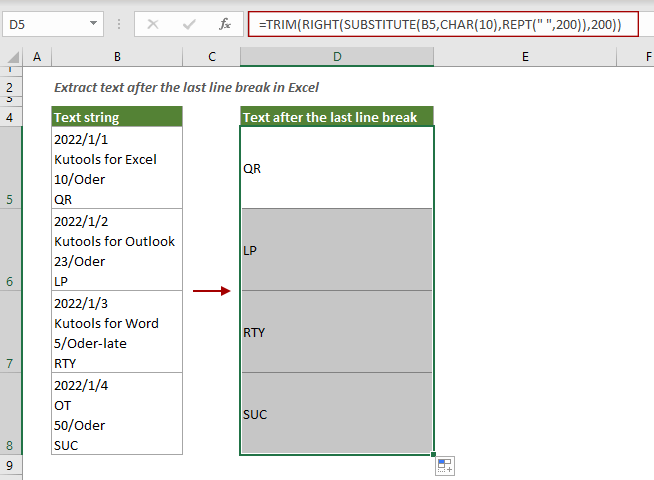Then the product no. part of each cell in the list is extracted as shown in the screenshot above.

Note: In the formula, CHAR(10) represents a line break on Windows.

2.7 Extract text before or after a word

In the previous parts, we have learned how to extract text before or after a character or delimiter. What should you do to extract text before or after an entire word? This section will introduce three methods to help you accomplish this task.

2.7.1 Extract text before a certain word with formula

The following formula helps you extract text before a certain word within a cell in Excel.

Generic formula

=IFERROR(LEFT(cell,FIND(word,cell)-1),cell)

Arguments

Cell: The cell from which you want to extract the text before a certain word.
Word: The word you want to extract all text before it. It can be a cell reference or an actual text string enclosed in double quotation marks;

Select a blank cell, enter the formula below and press the Enter key to get the result. Select this result cell and then drag its AutoFill Handle down to apply this formula to other cells.

In this example, we are going to extract all texts before the word “Excel”, so we directly type the word in the formula and enclose it in double quotes. Or you can reference to a cell that contains the word “Excel”.

=IFERROR(LEFT(B5,FIND("Excel",B5)-1),B5)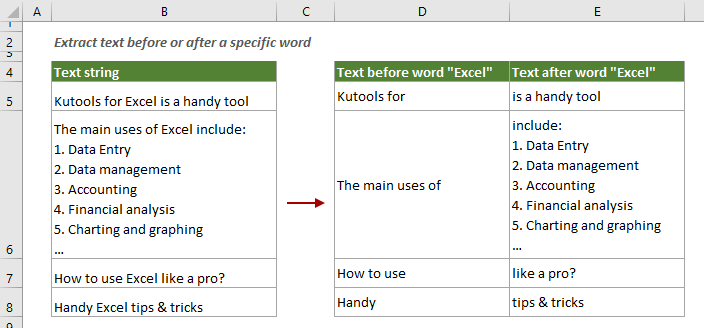Notes:

1) This formula is case-sensitive.
2) If the word you entered has duplicates in the same cell, the formula only extracts the text after the first occurrence.

2.7.2 Extract text after a certain word with formula

To extract text after a certain word, you can apply the following formula to get it done.

Generic formula

=TRIM(MID(cell,SEARCH(word,cell)+LEN(word),255))

Arguments

Cell: The cell from which you want to extract the text after a certain word.
Word: The word you want to extract all text after it. It can be a cell reference or an actual text string enclosed in double quotation marks;

Select a cell, enter the formula below and press the Enter key to get the result. Select the result cell and then drag its AutoFill Handle down to apply this formula to other cells.

=TRIM(MID(B5,SEARCH("Excel",B5)+LEN("Excel"),255))

Then you can see all texts after the word “Excel” in each cell are extracted as shown in the screenshot below.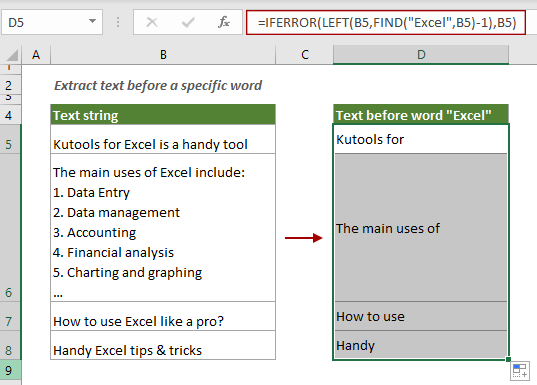Notes:

1) This formula is case-insensitive.
2) If the word you entered has duplicates in the same cell, the formula only extracts the text after the first occurrence.

2.7.3 Extract text before or after a certain word with an amazing tool

If you feel that using formula may cause a lot of inconvenience, here highly recommends the Extract Text utility of Kutools for Excel. This feature helps to automate the Extraction task in Excel with just a few clicks.

1. Click Kutools > Text > Extract Text to enable this feature.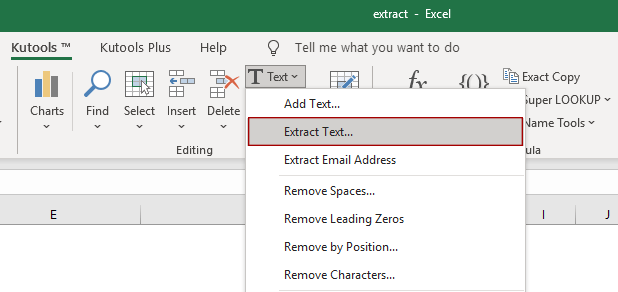2. In the Extract Text dialog box, do the following settings.

2.1) Make sure that you are in the Extract by location tab;
2.2) In the Range box, click the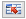button to select the range of cells where you want to extract texts;
2.3) In the Options section:
To extract all texts before a word, choose the before the text radio button and then enter the word into the text box;
To extract all texts after a word, choose the after the text radio button and then enter the word into the text box.
2.4) Click the OK button. See screenshot: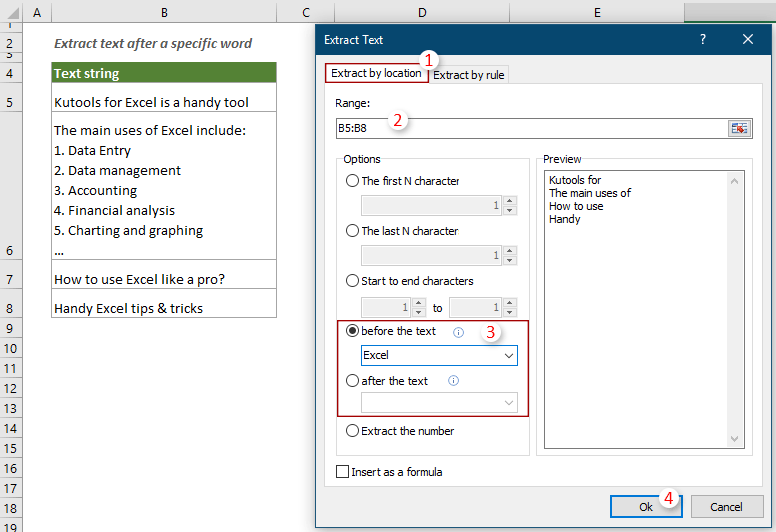Notes: If you want to create dynamic results, check the Insert as a formula box. Then the results will automatically update when the data in the range changes.

3. Then an Extract Text dialog box pops up, you need to select a cell to output the result and then click the OK button.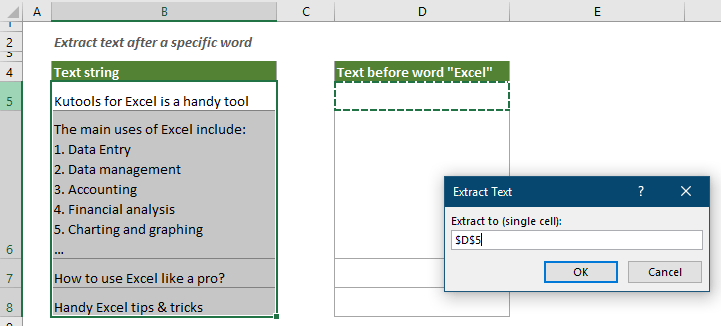Then texts before or after a certain word within each cell in the selected range are extracted immediately.

Note: This feature is case-sensitive.

If you want to have a free trial (30-day) of this utility, please click to download it, and then go to apply the operation according above steps.

##### 3. Extract between characters/words

If you want to extract text between certain characters or words, try the following methods.

3.1 Extract text between two characters

To extract text between two characters, which may be the same or different characters. This section provides several methods, and you can choose one of them according to your needs.

3.1.1 Extract text between two same characters with formula

As shown in the screenshot below, there is a list of text strings in column B, and you want to extract the number part between characters “/” from each cell in the range, the following formula can do you a favor.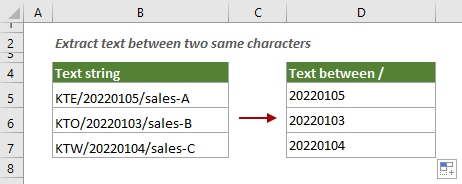Select a blank cell, copy or enter the formula below and press the Enter key to get the result. Select the result cell and then drag it AutoFill Handle down to get results of other cells in the list.

=SUBSTITUTE(MID(SUBSTITUTE("/" & B5&REPT(" ",6),"/",REPT(",",255)),2*255,255),",","")

Then the text between two same characters “/” is extracted from each cell in the range. See screenshot: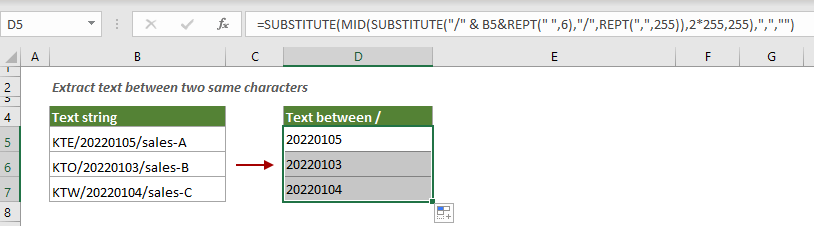Note:

1) B5 is the cell which you want to extract text between two same characters from;
2) “/” are two same characters you want to extract text between them.
You need to change these variables based on your own data.

3.1.2 Extract text between two different characters with formula

After learning how to extract text between two same characters within a cell, here we will demonstrate a formula to extract text between two different characters. As shown in the screenshot below, to extract only the email address between the “<” and “>” from each cell in column B, you can do as follows.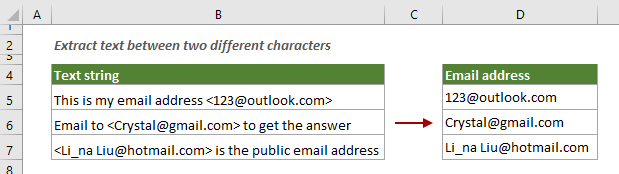Generic formula

=MID(LEFT(cel,FIND("end_char",cell)-1),FIND("start_char",cell)+1,LEN(cell))

Arguments

Cell: The cell from which you want to extract the text between two different characters;
End_char: The end character of the two different characters;
Start_char: The start character of the two different characters.

Select a blank cell, copy or enter the formula below and press the Enter key to get the result. Select this result cell and then drag its AutoFill Handle down to apply this formula to other cells.

=MID(LEFT(B5,FIND(">",B5)-1),FIND("<",B5)+1,LEN(B5))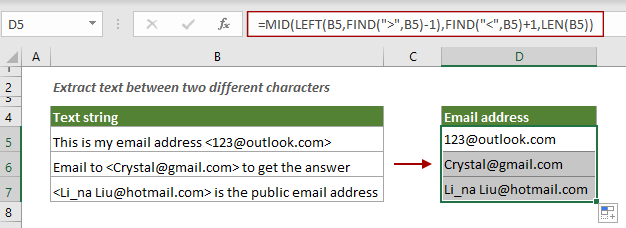You can see that only the text between the specified characters is extracted as shown in the screenshot above.

3.1.3 Extract text between two characters with an amazing tool

Here highly recommends the Extract strings between specified text feature of Kutools for Excel to help you easily extract text between two same or different characters within a cell in Excel.

1. Select a blank cell to output the result, and then click Kutools > Formula Helper > Formula Helper.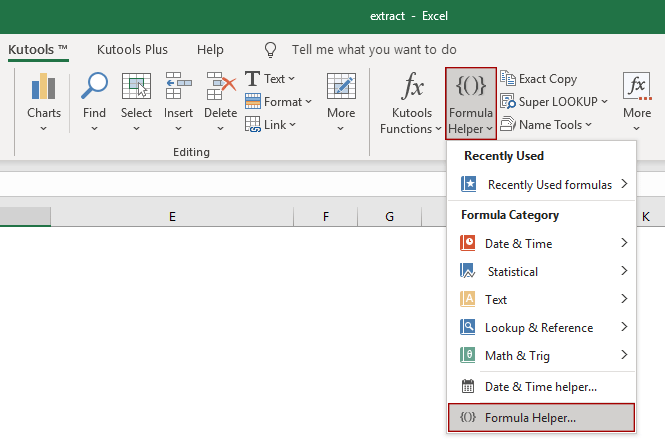2. In the Formulas Helper dialog box, do the following settings.

2.1) Check the Filter box and then enter the word “Extract” into the text box;
2.2) In the Choose a formula list box, click the Extract strings between specified text option;
2.3) In the Arguments input section:
In the Cell box, select a cell where you want to extract the text (here I select cell B5);
In the Start char(s) box, enter the start character of the two different characters;
In the End char(s) box, enter the end character of the two different characters.
2.4) Click Ok. See screenshot: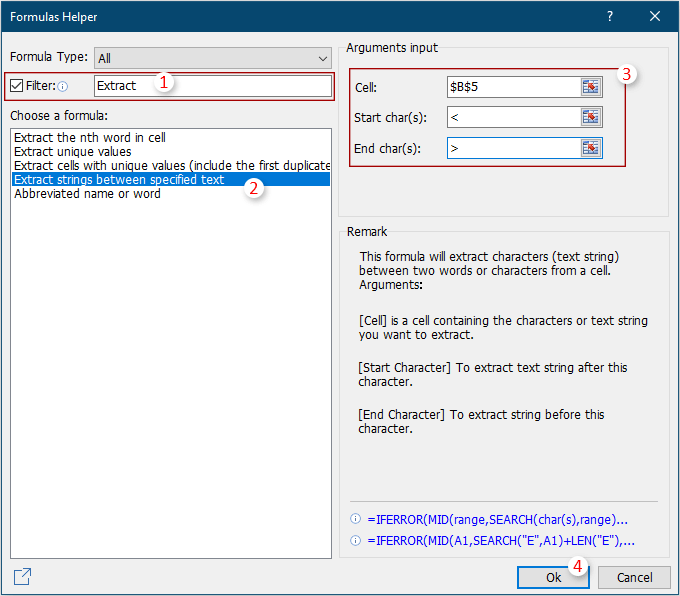3. Then only the text between “<” and “>” within the cell B5 is extracted. Meanwhile, a formula has been created, you can select this result cell and then drag its AutoFill Handle down to extract texts from other cells in the same list.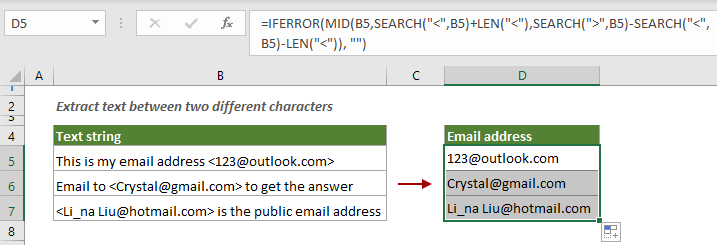If you want to have a free trial (30-day) of this utility, please click to download it, and then go to apply the operation according above steps.

3.1.4 Extract text between two characters (including the characters) by rule

If you want to keep the two characters after extracting, try to apply a rule in the Extract Text feature of Kutools for Excel.

1. Click Kutools > Text > Extract Text.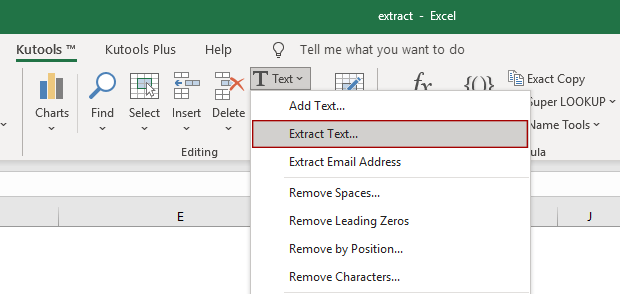2. In the Extract Text dialog box, do the following settings.

2.1) Click the Extract by rule tab;
2.2) In the Range section, click thebutton to select the range of cells where you want to extract the text between characters;
2.3) In the Text box, enter <*>
Tips:<” and “>” are the characters you want to extract text between them, * is a wildcard that represents any number of characters. You can enter the condition according to your needs.
2.4) Click the Add button to add the condition to the Rule description list box;
2.5) Click the Ok button. See screenshot: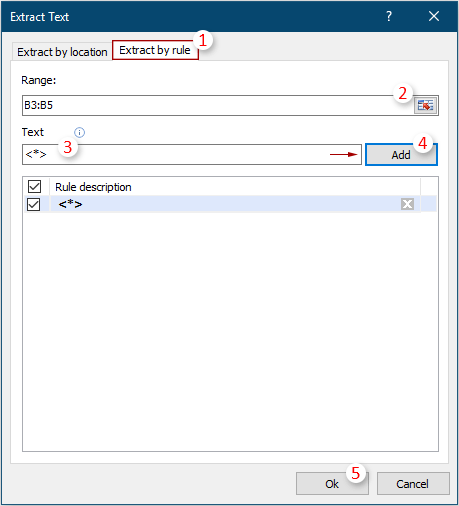3. Another Extract Text dialog box pops up, please select a cell to output the result, and then click the OK button.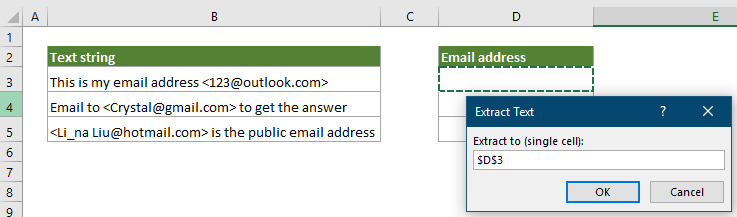Then the text between specified characters (including the characters) are extracted from each cell in the selected range in bulk.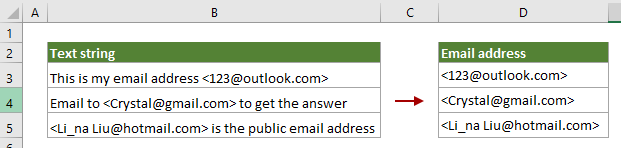If you want to have a free trial (30-day) of this utility, please click to download it, and then go to apply the operation according above steps.

3.2 Extract text between two words

In addition to extracting text between two characters, you may also need to extract text between two words. For example, extract all text strings between two words “KTE” and “feature” from each cell in column B as shown in the screenshot below. You can try one of the following methods to get it done.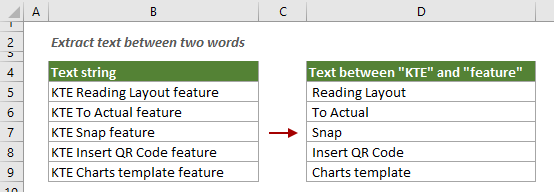3.2.1 Extract text between two words with formula

You can use a formula based on the MID function and the SEARCH function to extract all text strings between two words within a cell.

Generic formula

=MID(cell,SEARCH("start_word",cell)+3,SEARCH("end_word",cell)-SEARCH("start_word",cell)-4)

Arguments

Cell: the cell from which you want to extract all text strings between two words;
Start_word: the start word of the two words you want to extract all text strings after it;
Length1: the character length of the start word.
End_word: the end word of the two words you want to extract all text strings before it.
Length2: the character length of the start word plus 1.

Select a blank cell, copy or enter the formula below and press the Enter key to get the result. Select this result cell and then drag its AutoFill Handle down to apply this formula to other cells.

=MID(B5,SEARCH("KTE",B5)+3,SEARCH("feature",B5)-SEARCH("KTE",B5)-4)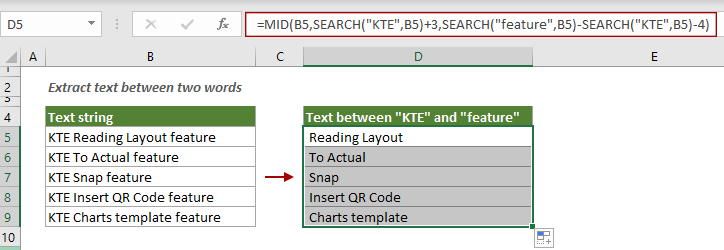Note: In the formula, the number 3 represents the character length of the word "KTE"; the number 4 represents the character length of the word "KTE" plus 1.

You can see all text strings between the specified two words are extracted from each cell in column B.

3.2.2 Extract text between two words with an amazing tool

For many Excel users, formulas may be hard to remember and handle. Here, with the Extract strings between specified text feature of Kutools for Excel, you can easily extract text between two words with just a few clicks.

1. Select a cell to output the result, and then click Kutools > Formula Helper > Formula Helper.2. In the Formula Helper dialog box, you need to configure as follows.

2.1) Check the Filter box and then enter the word “Extract” into the text box;
2.2) In the Choose a formula list box, click the Extract strings between specified text option;
2.3) In the Arguments input section:
In the Cell box, select a cell where you want to extract the text (here I select cell B5);
In the Start char(s) box, enter the start word of the two words you want to extract all text strings after it;
In the End char(s) box, enter the end word of the two words you want to extract all text strings before it.
2.4) Click Ok. See screenshot: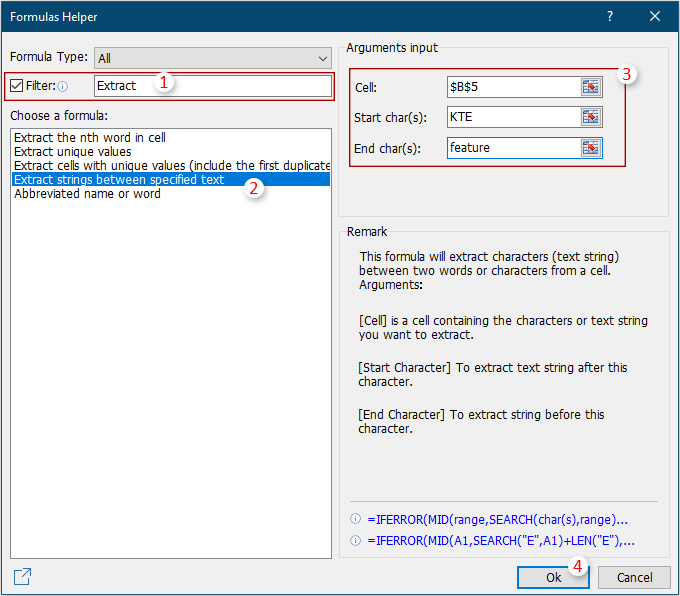3. Then all text strings between two words “KTE” and “feature” within the cell B5 is extracted. Meanwhile, a formula has been created, you can select this result cell and then drag its AutoFill Handle down to extract texts from other cells in the same list.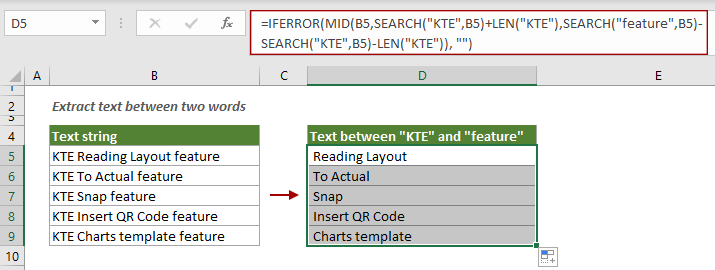If you want to have a free trial (30-day) of this utility, please click to download it, and then go to apply the operation according above steps.

#### Extract numbers by position

For a list of alphanumeric strings, there may be three cases:

1. The number is at the beginning of the text;
2. The number is at the end of the text;
3. The number can be anywhere in the text.

In this section, we will provide different methods that can be used to extract the numbers in each case that mentioned above.

##### 1 Extract number from the left of a string

This part will introduce a formula to help you extract only numbers that appear before text in a cell.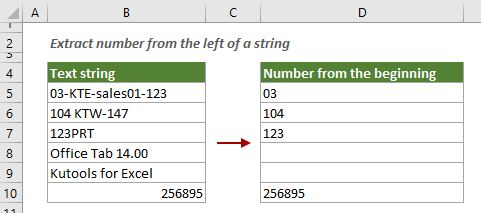Generic formula

=LEFT(cell, MATCH(FALSE, ISNUMBER(MID(cell, ROW(INDIRECT("1:"&LEN(cell)+1)), 1) *1), 0) -1)

Arguments

Cell: The cell from which you want to extract number at the left of text string.

Note:

1) If you are using Excel 2019 and earlier, you need to press the Ctrl + Shift + Enter keys to confirm this array formula.
2) If you are using Excel 365 or Excel 2021, just confirm this formula with the Enter key.

Select a blank cell, enter the formula below and press Ctrl + Shift + Enter or Enter key to get the result. Select this result cell and then drag its AutoFill Handle down to get numbers of other cells.

=LEFT(B5, MATCH(FALSE, ISNUMBER(MID(B5, ROW(INDIRECT("1:"&LEN(B5)+1)), 1) *1), 0) -1)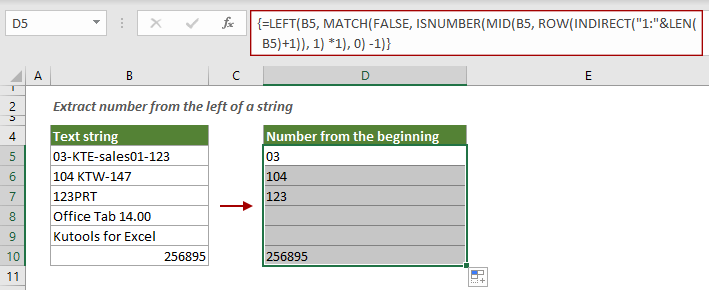Notes:

1) If a cell contains only numbers, the whole number will be extracted.
2) This formula only extracts the numbers at the left of a text string. If numbers exist in the middle or at the end of the text string, they will be ignored.

##### 2 Extract number from the right of a string

As shown in the screenshot below, to extract only the numbers that appear after text in a cell, try the following formula.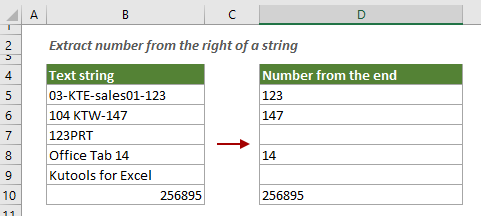Generic formula

=RIGHT(cell, LEN(cell) - MAX(IF(ISNUMBER(MID(cell, ROW(INDIRECT("1:"&LEN(cell))), 1) *1)=FALSE, ROW(INDIRECT("1:"&LEN(cell))), 0)))

Arguments

Cell: The cell from which you want to extract number from the beginning of text string.

Note:

1) If you are using Excel 2019 and earlier, you need to press the Ctrl + Shift + Enter keys to confirm this array formula.
2) If you are using Excel 365 or Excel 2021, just confirm this formula with the Enter key.

Select a blank cell, enter the formula below and press Ctrl + Shift + Enter or Enter key to get the result. Select this result cell and then drag its AutoFill Handle down to get numbers of other cells.

=RIGHT(B5, LEN(B5) - MAX(IF(ISNUMBER(MID(B5, ROW(INDIRECT("1:"&LEN(B5))), 1) *1)=FALSE, ROW(INDIRECT("1:"&LEN(B5))), 0)))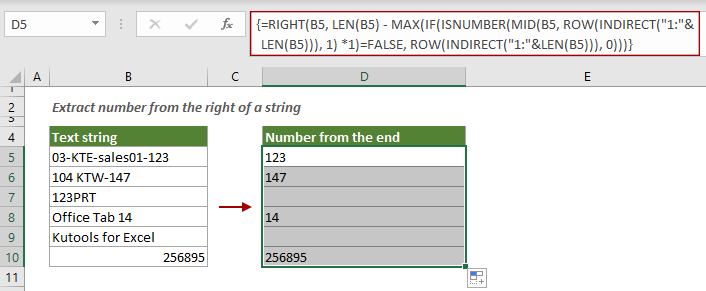Notes:

1) If a cell contains only numbers, the whole number will be extracted.
2) This formula only extracts the numbers from the right of a text string. If numbers exist in the middle or beginning of the text string, they will be ignored.

##### 3. Extract all numbers from any position in a text string

The methods above help to extract number only from the left or right of a text string. If you want to extract all numbers from anywhere in a text string, here we provide three methods for you to get it done.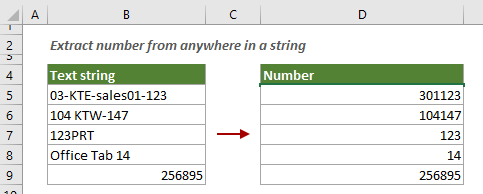3.1 Extract all numbers from anywhere in a string with formula

You can apply the following formula to extract all numbers from anywhere in a text string in Excel.

1. Select a blank cell, copy or enter the formula below and press the Enter key to get all numbers from the cell B5.

=SUMPRODUCT(MID(0&B5, LARGE(INDEX(ISNUMBER(--MID(B5, ROW(INDIRECT("1:"&LEN(B5))), 1)) * ROW(INDIRECT("1:"&LEN(B5))), 0), ROW(INDIRECT("1:"&LEN(B5))))+1, 1) * 10^ROW(INDIRECT("1:"&LEN(B5)))/10)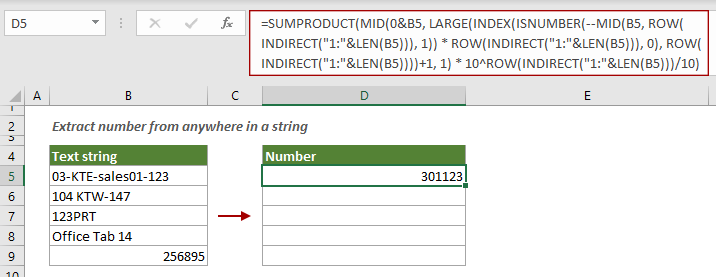2. Select the result cell and then drag its AutoFill Handle down to get all numbers of other cells.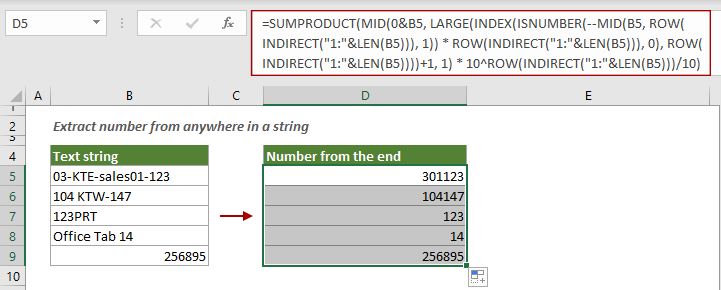3.2 Extract all numbers from anywhere in a string with VBA

The formula above is too long and complicated for many Excel users. Actually, you can run a VBA script to automate the task in Excel. You can do as follows.

1. Press the Alt + F11 keys to open the Microsoft Visual Basic for Applications window.

2. In the opening Microsoft Visual Basic for Applications window, click Insert > Module. Then copy the VBA below into the Module code window.

VBA code: Extract all numbers from anywhere in a text string

``````Sub ExtrNumbersFromRange()
'Updated by Extendoffice 20220106
Dim xRg As Range
Dim xDRg As Range
Dim xRRg As Range
Dim nCellLength As Integer
Dim xNumber As Integer
Dim strNumber As String
Dim xTitleId As String
Dim xI As Integer
xTitleId = "KutoolsforExcel"
Set xDRg = Application.InputBox("Please select text strings:", xTitleId, "", Type:=8)
If TypeName(xDRg) = "Nothing" Then Exit Sub
Set xRRg = Application.InputBox("Please select output cell:", xTitleId, "", Type:=8)
If TypeName(xRRg) = "Nothing" Then Exit Sub
xI = 0
strNumber = ""
For Each xRg In xDRg
xI = xI + 1
nCellLength = Len(xRg)
For xNumber = 1 To nCellLength
If IsNumeric(Mid(xRg, xNumber, 1)) Then
strNumber = strNumber & Mid(xRg, xNumber, 1)
End If
Next xNumber
xRRg.Item(xI) = strNumber
strNumber = ""
Next xRg
End Sub``````

3. Press the F5 key to run the code. In the opening KutoolsforExcel dialog box, select the range of cells where you want to extract all numbers from each cell, and then click the OK button.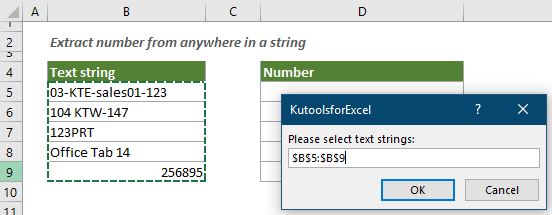4. Then another KutoolsforExcel dialog box pops up. In this dialog box, select a destination cell and click OK.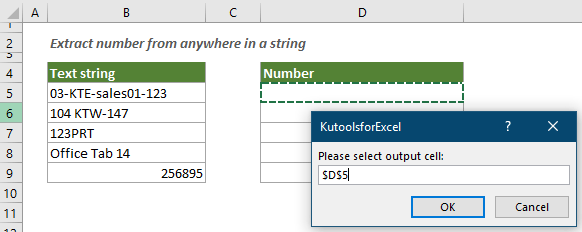Then all numbers are extracted from each cell in the selected range in bulk.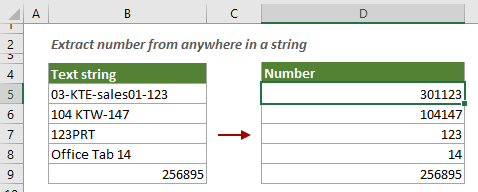##### 4. Extract numbers after specific text

As shown in the screenshot below, to extract any numbers after the specific text “No.”, this section provides two methods to help you get it done.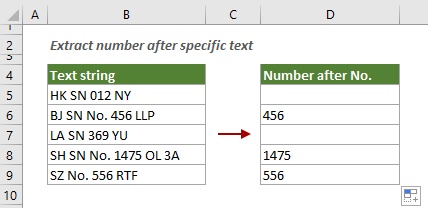4.1 Extract numbers after a specific text with formula

You can apply the following formula to extract numbers after a specific text in a cell in Excel.

Generic formula:

=LOOKUP(10^6,1*MID(cell,MIN(FIND({0,1,2,3,4,5,6,7,8,9},cell&"0123456789",FIND("text"," "&cell&" "))),{2,3,4,5,6}))

Arguments

Cell: The cell from which you want to extract numbers after a specific text;
Text: The text you want to extract numbers after it.

Select a blank cell, copy or enter the formula below and press the Enter key to get the result. Select this result cell and then drag its AutoFill Handle down to apply this formula to other cells.

=LOOKUP(10^6,1*MID(B5,MIN(FIND({0,1,2,3,4,5,6,7,8,9},B5&"0123456789",FIND("No."," "&B5&" "))),{2,3,4,5,6}))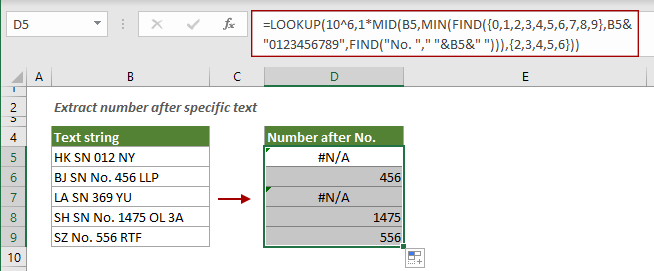Notes:

1) If a cell does not contain the specific text, the formula will return #N/A.
2) The formula is case-sensitive.

4.2 Extract numbers after a specific text with user-defined function

The following user-defined function can also help to extract numbers after a specific text in a cell. Please do as follows.

1. Press the Alt + F11 keys to open the Microsoft Visual Basic for Applications window.

2. In the Microsoft Visual Basic for Applications window, click Insert > Module, and then copy the VBA code below into the Module code window.

VBA code: extract numbers after a specific text in a cell

``````Function GetNumberAfterTheChar(Rng As Range, Char As String)
'Updated by Extendoffice 20220106
Dim xValue As String
Dim xRntString As String
Dim xStart As Integer
Dim xC
xValue = Rng.Text
xStart = InStr(1, xValue, Char, vbTextCompare)
If IsEmpty(xStart) Then
GetNumberAfterTheChar = ""
Exit Function
End If
If xStart < 1 Then
GetNumberAfterTheChar = ""
Exit Function
End If
xStart = xStart - 1 + Len(Char)
If xStart < 1 Then
GetNumberAfterTheChar = ""
Exit Function
End If
xValue = Mid(xValue, xStart + 1)
xRntString = ""
For xI = 1 To Len(xValue)
xC = Mid(xValue, xI, 1)
Select Case Asc(xC)
Case 48 To 57
xRntString = xRntString & xC
Case Else
Exit For
End Select
Next
GetNumberAfterTheChar = xRntString
End Function``````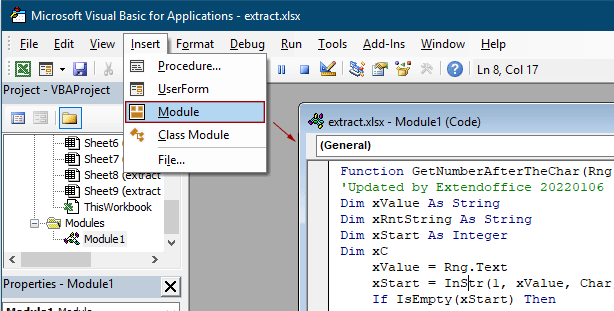3. Press the Alt + Q keys to close the Microsoft Visual Basic for Applications window.

4. Select a cell, enter the formula below and press the Enter key. Select this result cell and then drag its AutoFill Handle down to apply this formula to other cells.

=GetNumberAfterTheChar(B5,"No. ")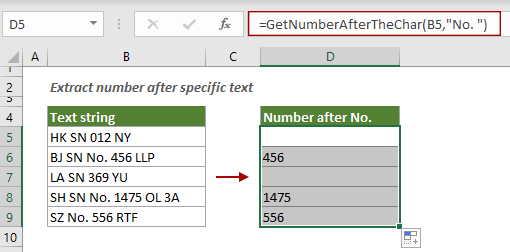Notes:

1) In this formula, B5 is the cell where you want to extract the number, and “No. ” is the specific text that you want to extract the number after it. You can change them as you need.
2) If there is a delimiter between the specific text and the number, please add the delimiter at the end of the text. In this case, I added a space after the text “No.”, which is finally displayed as “No. ”.
3) This method is case-insensitive;
4) If a cell does not contain the specific text, the formula will return a blank result.

#### Related articles:

Excel Tutorial: Split Text, Number, And Date Cells (Separate Into Multiple Columns)
This tutorial is divided into three parts: split text cells, split number cells and split date cells. Each part provides different examples to help you know how to handle the splitting job when encountering the same problem.
Click to know more...

Excel Add Text And Number Into Specified Position Of Cell
In Excel, adding texts or numbers to cells is a very common job. Such as adding space between names, adding prefix or suffix to cells, adding dashes to social numbers. Here in this tutorial, it lists almost all of adding scenarios in Excel and provides the corresponding methods for you.
Click to know more...

Excel Remove Characters, Words, Numbers From Text Strings
Supposing, you have a long list of text strings which contain characters, numbers or other specific symbols. In certain case, you may need to remove some characters based on position, such as from right, left or middle from the text strings, or delete some unwanted characters, numbers from the list of strings. Finding the solutions one by one will get you a headache, this tutorial collects all kinds of methods for removing characters, words or numbers in Excel.
Click to know more...

### Best Office Productivity Tools

 Popular Features: Find, Highlight or Identify Duplicates   |  Delete Blank Rows   |  Combine Columns or Cells without Losing Data   |   Round without Formula ... Super Lookup: Multiple Criteria VLookup  |   Multiple Value VLookup  |   VLookup Across Multiple Sheets   |   Fuzzy Lookup .... Advanced Drop-down List: Quickly Create Drop Down List   |  Dependent Drop Down List   |  Multi-select Drop Down List .... Column Manager: Add a Specific Number of Columns  |  Move Columns  |  Toggle Visibility Status of Hidden Columns  |  Compare Ranges & Columns ... Featured Features: Grid Focus   |  Design View   |   Big Formula Bar   |  Workbook & Sheet Manager   |  Resource Library (Auto Text)   |  Date Picker   |  Combine Worksheets   |  Encrypt/Decrypt Cells   |  Send Emails by List   |  Super Filter   |   Special Filter (filter bold/italic/strikethrough...) ... Top 15 Toolsets:  12 Text Tools (Add Text, Remove Characters, ...)   |   50+ Chart Types (Gantt Chart, ...)   |   40+ Practical Formulas (Calculate age based on birthday, ...)   |   19 Insertion Tools (Insert QR Code, Insert Picture from Path, ...)   |   12 Conversion Tools (Numbers to Words, Currency Conversion, ...)   |   7 Merge & Split Tools (Advanced Combine Rows, Split Cells, ...)   |   ... and more

Supercharge Your Excel Skills with Kutools for Excel, and Experience Efficiency Like Never Before. Kutools for Excel Offers Over 300 Advanced Features to Boost Productivity and Save Time.  Click Here to Get The Feature You Need The Most...#### Office Tab Brings Tabbed interface to Office, and Make Your Work Much Easier

• Enable tabbed editing and reading in Word, Excel, PowerPoint, Publisher, Access, Visio and Project.
• Open and create multiple documents in new tabs of the same window, rather than in new windows.
• Increases your productivity by 50%, and reduces hundreds of mouse clicks for you every day!